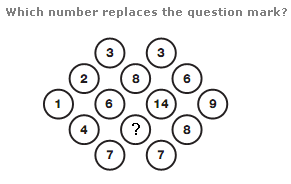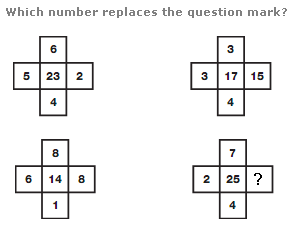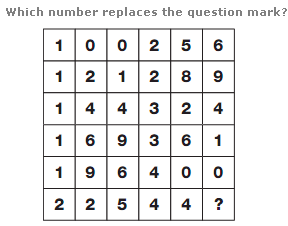# Puzzles - Number puzzles

### Exercise :: Number puzzlesAnswer : 12 Explanation : The diagram contains two diamonds of 3x3 circles, arranged side by side, with the central 4 circles overlapping. Starting in the central left circle of each diamond, the numbers from 1 to 9 are written in lines, moving up and to the right. In the circles where the diamonds overlap, the values are added together to give the ones shown on the final diagram.Answer : 1 Explanation : Add up the four outer numbers and place your answer in the centre square of the shape one place clockwise.Answer : 1 Explanation : Split the grid in half vertically, to give two columns, 3 squares wide. Working from top to bottom, left then right, and reading each 3 square row as a whole 3 digit number, the columns follow the sequence of square numbers, from 10 to 21.